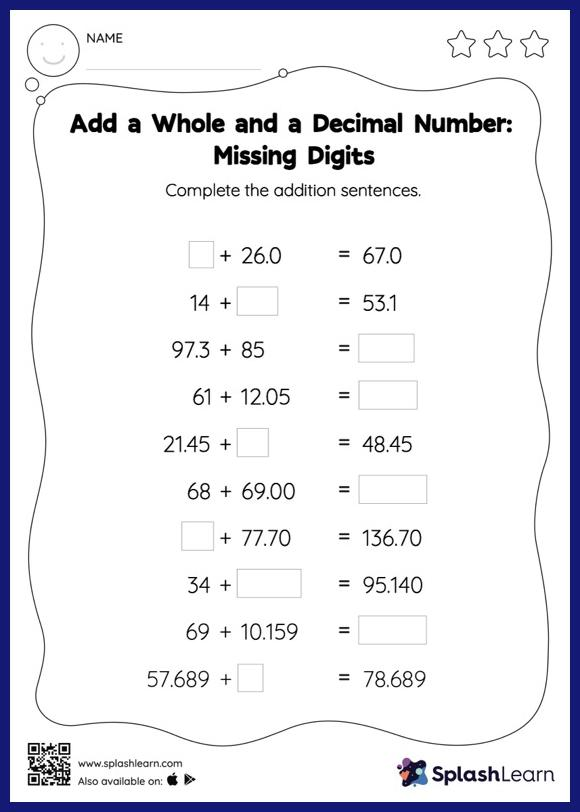# Add a Whole and a Decimal Number: Missing Numbers Worksheet

Home > Add a Whole and a Decimal Number: Missing NumbersHelp your little one develop a knack for math with this add a whole and a decimal number worksheet. When adding decimals on this worksheet, students align the decimal points and use zero as a placeholder. Then to find the missing number in the add a whole and a decimal number worksheet, students then apply the relationship between addition and subtraction.